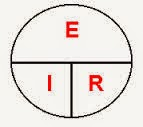## Sunday, August 10, 2014

### Post-Modern Ohm's Law AbbreviationsWhen I was growing up, this was symbol that helped us remember Ohm's law.  "E" is for electromotive force (EMF) in volts.  "I" is for intensity of charge motion or current in amperes.  And "R" is for resistance in ohms.

The symbol works this way: To determine voltage in a circuit, put your thumb over the E and you are reminded that EMF in volts equals the intensity of charge motion in amps multiplied by resistance in ohms.  To determine current ("I"), put your thumb over the I and you see that amps equals the voltage divided by the resistance.  To determine resistance, cover R and you are reminded that EMF divided by intensity of charge (or volts by amperes or amps) will get you get the resistance (that is, the ohms).

Pretty neat, except that since I learned Ohm's law, post-modern electrical engineering whippersnappers have been changing the abbreviations.  Now for E, we may get V in an Ohm's law formula discussion.  That may seem more appropriate, as V of course stands for volt.  But E, by referring to electromotive force, reminds you of the signal characteristic of voltage.

Similarly, we now get C instead of I for current.  Maybe this, like V for voltage, is an obvious improvement.  But it is not simply current that kills you, it is the intensity of charge motion that will do it.  Every electrical impulse has current, for Pete's sake.  Not every one of them has 1000 amps.  It is probably good to remember that when dealing with electrical circuits.